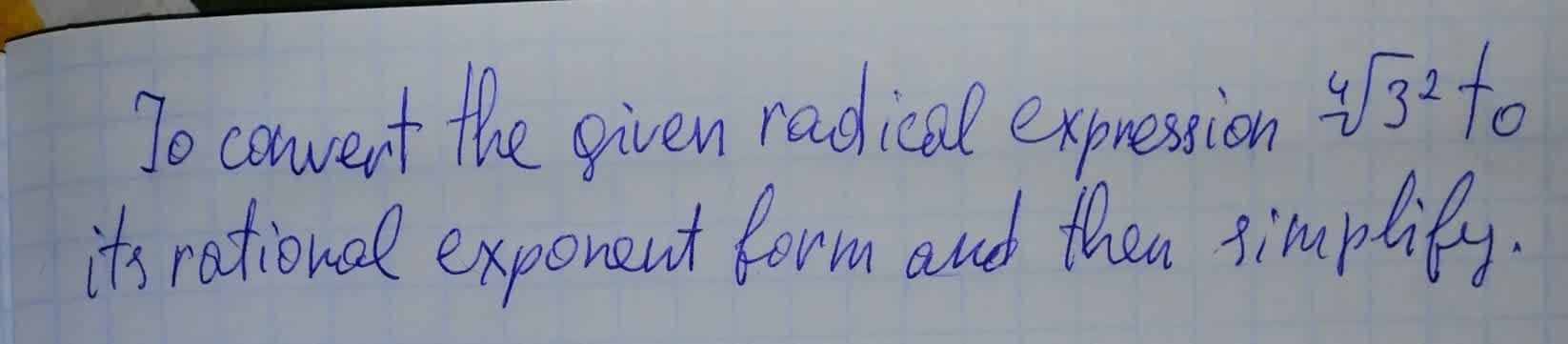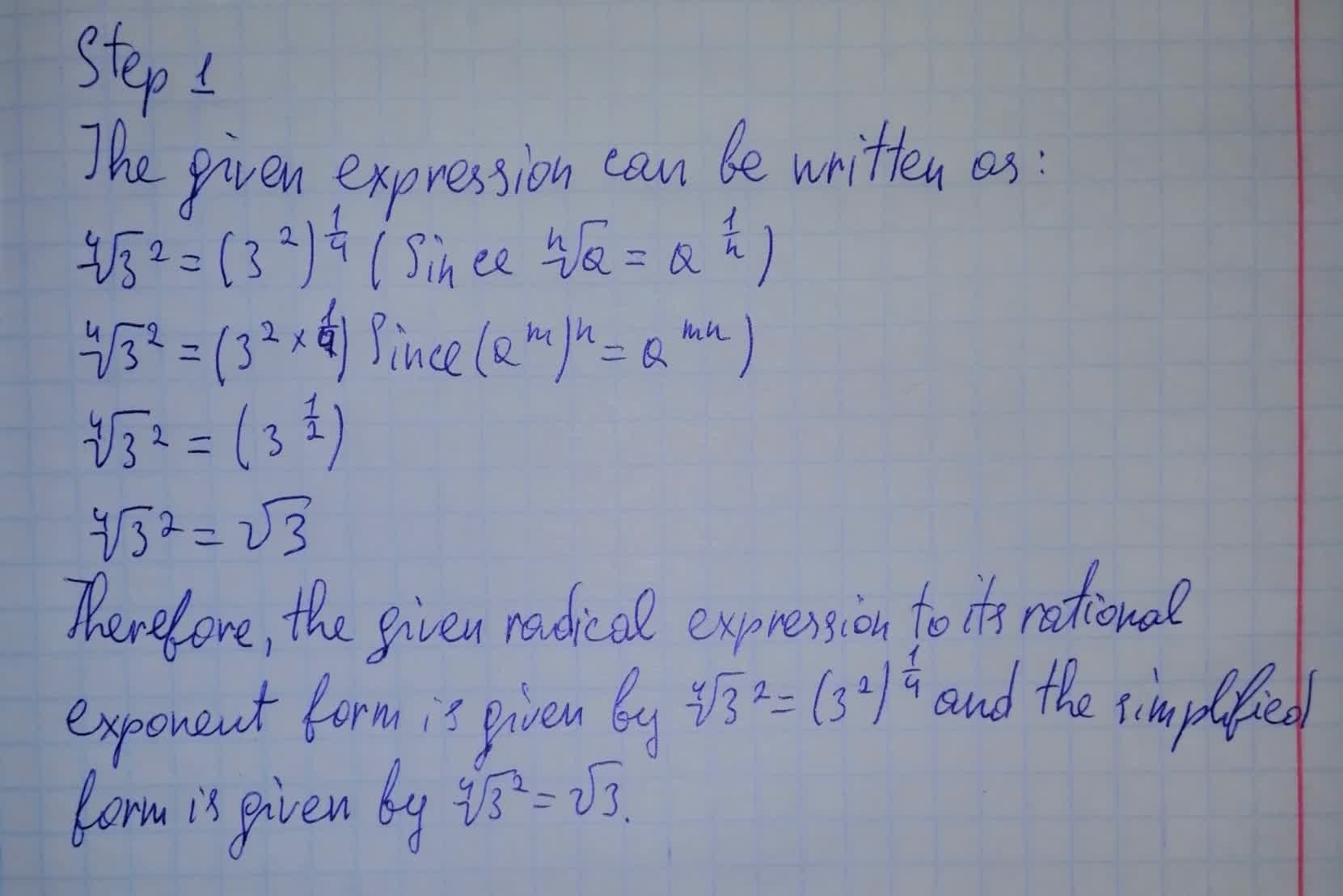Question# To convert the given radical expression \sqrt{3^2} to its rational exponent form and then simplify

Conic sections
ANSWEREDTo convert the given radical expression $$\sqrt{3^2}$$ to its rational exponent form and then simplify